# Creating plots in R using ggplot2 - part 1: line plots

written in

I teamed up with Mauricio Vargas Sepúlveda about a year ago to create some graphing tutorials in R. On this blog you will find a series of tutorials on how to use the ggplot2 package to create beautiful and informative data visualisations. Each tutorial will explain how to create a different type of plot, and will take you step-by-step from a basic plot to a highly customised graph.

In this first tutorial, we will demonstrate some of the many options the ggplot2 package has for creating and customising line plots. We will use an international trade dataset made by ourselves from different sources (Chile Customs, Central Bank of Chile and General Directorate of International Economic Relations).

Mauricio and I have also published these graphing posts as a book on Leanpub. We tend to put any changes or updates to the code in the book before these blog posts, so please check it out if you have any issues with the code examples in this post; otherwise feel free to contact us with any questions!

The first thing to do is load in the data and libraries, as below:

library(ggplot2)
library(ggthemes)
library(extrafont)



In this tutorial, we will work towards creating the line plot below. We will take you from a basic line plot and explain all the customisations we add to the code step-by-step.## Basic graph

In order to initialise a plot we tell ggplot that charts.data is our data, and specify the variables on each axis. We then instruct ggplot to render this as a line plot by adding the geom_line command.

p1 <- ggplot() + geom_line(aes(y = export, x = year, colour = product),
data = charts.data, stat="identity")
p1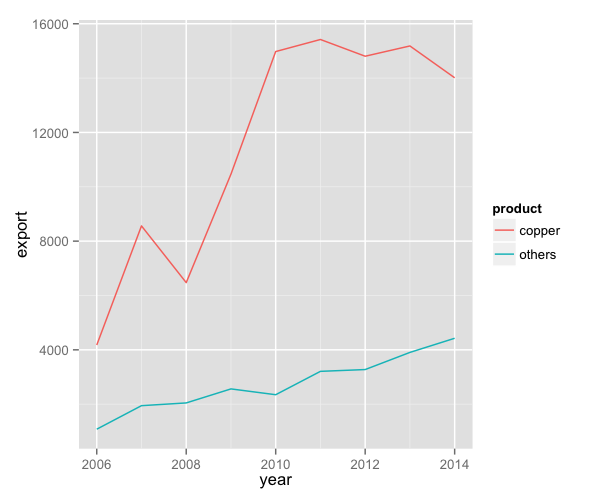To change the line width, we add a size argument to geom_line.

p1 <- ggplot() + geom_line(aes(y = export, x = year, colour = product), size=1.5,
data = charts.data, stat="identity")
p1## Changing variables display

To change the variables displayed name, we need to re-factor our data labels in charts.data data frame. Then we move the legend to the bottom using the theme command.

charts.data <- as.data.frame(charts.data)
charts.data$product <- factor(charts.data$product, levels = c("copper","others"),
labels = c("Copper","Pulp wood, Fruit, Salmon & Others"))

p1 <- ggplot() + geom_line(aes(y = export, x = year, colour = product), size=1.5,
data = charts.data, stat="identity") +
theme(legend.position="bottom", legend.direction="horizontal", legend.title = element_blank())
p1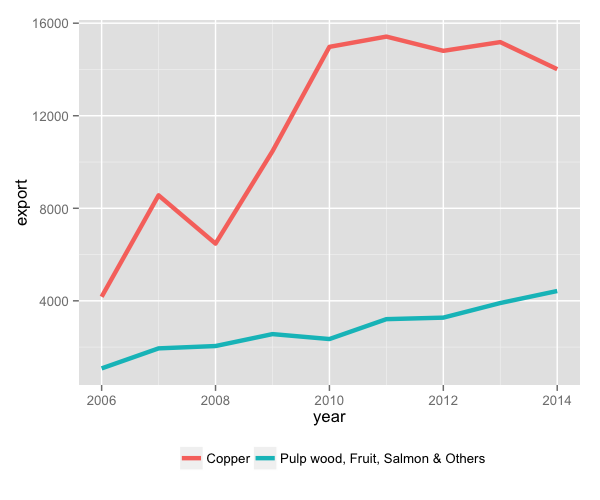To change the axis tick marks, we use the scale_x_continuous and/or scale_y_continuous commands.

p1 <- p1 + scale_x_continuous(breaks=seq(2006,2014,1))
p1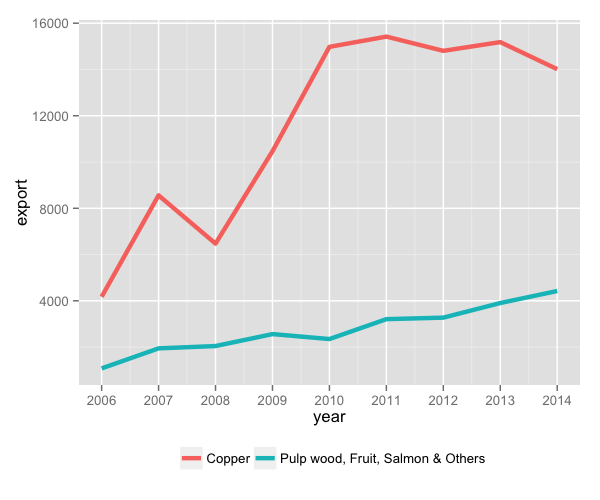To add a title, we include the option ggtitle and include the name of the graph as a string argument, and to change the axis names we use the labs command.

p1 <- p1 + ggtitle("Composition of Exports to China ($)") + labs(x="Year", y="USD million") p1## Adjusting color palette To change the colours, we use the scale_colour_manual command. colour <- c("#5F9EA0", "#E1B378") p1 <- p1 + scale_colour_manual(values=colour) p1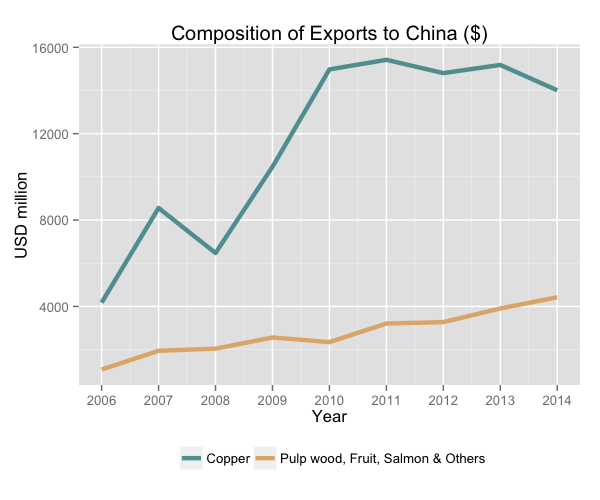## Using the white theme We’ll start using a simple theme customisation made adding theme_bw() after ggplot(). That theme argument can be modified to use different themes. p1 <- ggplot() + theme_bw() + geom_line(aes(y = export, x = year, colour = product), size=1.5, data = charts.data, stat="identity") + theme(legend.position="bottom", legend.direction="horizontal", legend.title = element_blank()) + scale_x_continuous(breaks=seq(2006,2014,1)) + labs(x="Year", y="USD million") + ggtitle("Composition of Exports to China ($)") +
scale_colour_manual(values=colour)
p1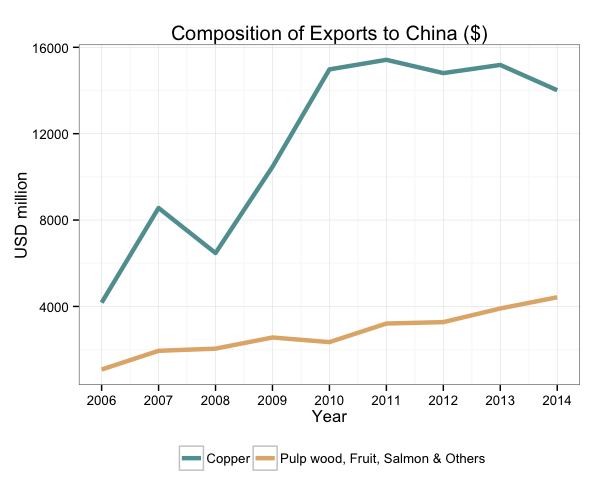## Creating an XKCD style chart

Of course, you may want to create your own themes as well. ggplot2 allows for a very high degree of customisation, including allowing you to use imported fonts. Below is an example of a theme Mauricio was able to create which mimics the visual style of XKCD. In order to create this chart, you first need to import the XKCD font, install it on your machine and load it into R using the extrafont package. These instructions are taken from here:

library(extrafont)

dest="xkcd.ttf", mode="wb")
system("mkdir ~/.fonts")
system("cp xkcd.ttf  ~/.fonts")
font_import(paths = "~/.fonts", pattern="[X/x]kcd")
fonts()


You can then create your graph:

fill <- c("#56B4E9", "#ff69b4")

p1 <- ggplot() +
geom_line(aes(y = export, x = year, colour = product), size=1.5, data = charts.data,
stat="identity") +
theme(legend.position="bottom", legend.direction="horizontal",
legend.title = element_blank()) +
scale_x_continuous(breaks=seq(2006,2014,1)) +
labs(x="Year", y="USD million") +
ggtitle("Composition of Exports to China ($)") + scale_color_manual(values=fill) + theme(axis.line = element_line(size=1, colour = "black"), panel.grid.major = element_blank(), panel.grid.minor = element_blank(), panel.border = element_blank(), panel.background = element_blank()) + theme(plot.title=element_text(family="xkcd-Regular"), text=element_text(family="xkcd-Regular"), axis.text.x=element_text(colour="black", size = 10), axis.text.y=element_text(colour="black", size = 10), legend.key=element_rect(fill="white", colour="white")) p1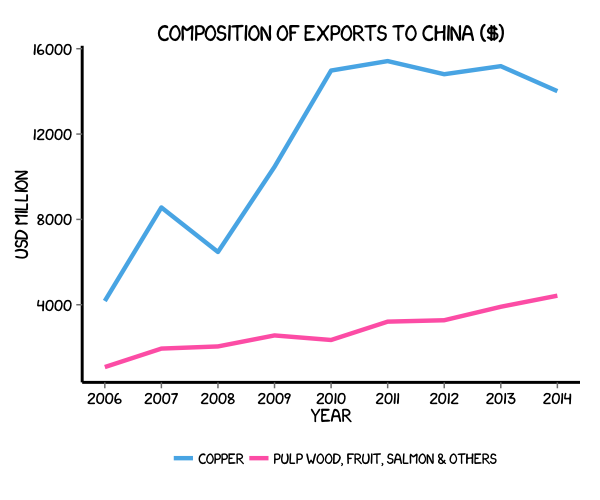## Using ‘The Economist’ theme There are a wider range of pre-built themes available as part of the ggthemes package (more information on these here). Below we’ve applied theme_economist(), which approximates graphs in the Economist magazine. It is also important that the font change argument inside theme is optional and it’s only to obtain a more similar result compared to the original. For an exact result you need ‘Officina Sans’ which is a commercial font and is available here. p1 <- ggplot() + theme_economist() + scale_colour_economist() + theme(plot.title=element_text(family="OfficinaSanITC-Book"), text=element_text(family="OfficinaSanITC-Book")) + geom_line(aes(y = export, x = year, colour = product), size=1.5, data = charts.data, stat="identity") + theme(legend.position="bottom", legend.direction="horizontal", legend.title = element_blank()) + scale_x_continuous(breaks=seq(2006,2014,1)) + labs(x="Year", y="USD million") + ggtitle("Composition of Exports to China ($)")
p1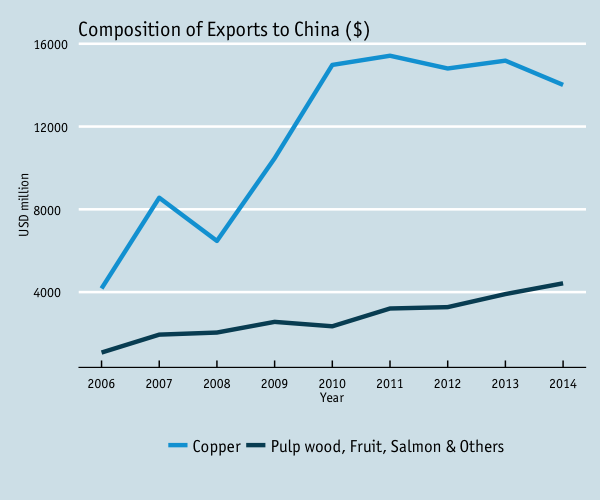## Using ‘Five Thirty Eight’ theme

Below we’ve applied theme_fivethirtyeight(), which approximates graphs in the nice FiveThirtyEight website. Again, it is also important that the font change is optional and it’s only to obtain a more similar result compared to the original. For an exact result you need ‘Atlas Grotesk’ which is a commercial font and is available here.

p1 <- ggplot() + theme_fivethirtyeight() + scale_colour_fivethirtyeight() +
theme(plot.title=element_text(family="Atlas Grotesk Medium"),
text=element_text(family="Atlas Grotesk Light")) +
geom_line(aes(y = export, x = year, colour = product), size=1.5, data = charts.data,
stat="identity") +
theme(legend.position="bottom", legend.direction="horizontal",
legend.title = element_blank()) +
scale_x_continuous(breaks=seq(2006,2014,1)) +
labs(x="Year", y="USD million") +
ggtitle("Composition of Exports to China ($)") p1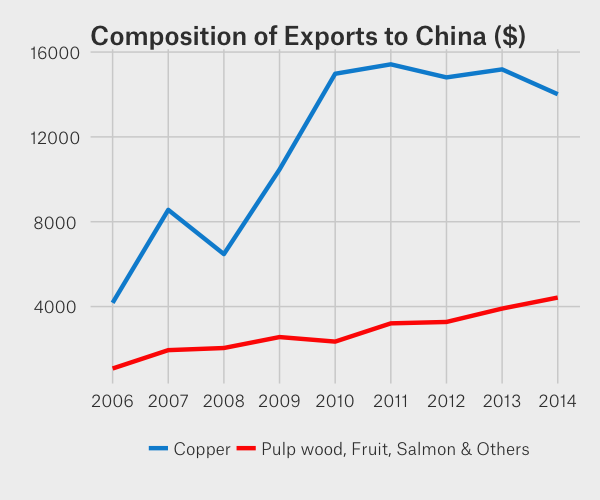## Creating your own theme As before, you can modify your plots a lot as ggplot2 allows many customisations. Here we present our original result shown at the top of page. colour <- c("#40b8d0", "#b2d183") p1 <- ggplot() + geom_line(aes(y = export, x = year, colour = product), size=1.5, data = charts.data, stat="identity") + theme(legend.position="bottom", legend.direction="horizontal", legend.title = element_blank()) + scale_x_continuous(breaks=seq(2006,2014,1)) + labs(x="Year", y="USD million") + ggtitle("Composition of Exports to China ($)") +
scale_colour_manual(values=colour) +
theme(axis.line = element_line(size=1, colour = "black"),
panel.grid.major = element_line(colour = "#d3d3d3"), panel.grid.minor = element_blank(),
panel.border = element_blank(), panel.background = element_blank()) +
theme(plot.title = element_text(size = 14, family = "Tahoma", face = "bold"),
text=element_text(family="Tahoma"),
axis.text.x=element_text(colour="black", size = 10),
axis.text.y=element_text(colour="black", size = 10),
legend.key=element_rect(fill="white", colour="white"))
p1